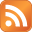# I-Ninja PS2 cheatsCheat codes

During the game, press start followed by one of these codes:

Hold R1, triangle, triangle, triangle, triangle, release R1, hold L1, triangle, triangle, hold R1 + L1, triangle, circle, triangle - Big heads
Hold R1, square, square, square, circle, release R1, hold L1, triangle, triangle, release L1, hold R1, square, square - Complete current mission
Hold L1 + R1, circle, square, circle, triangle, triangle, square, circle, square - Sword upgrade